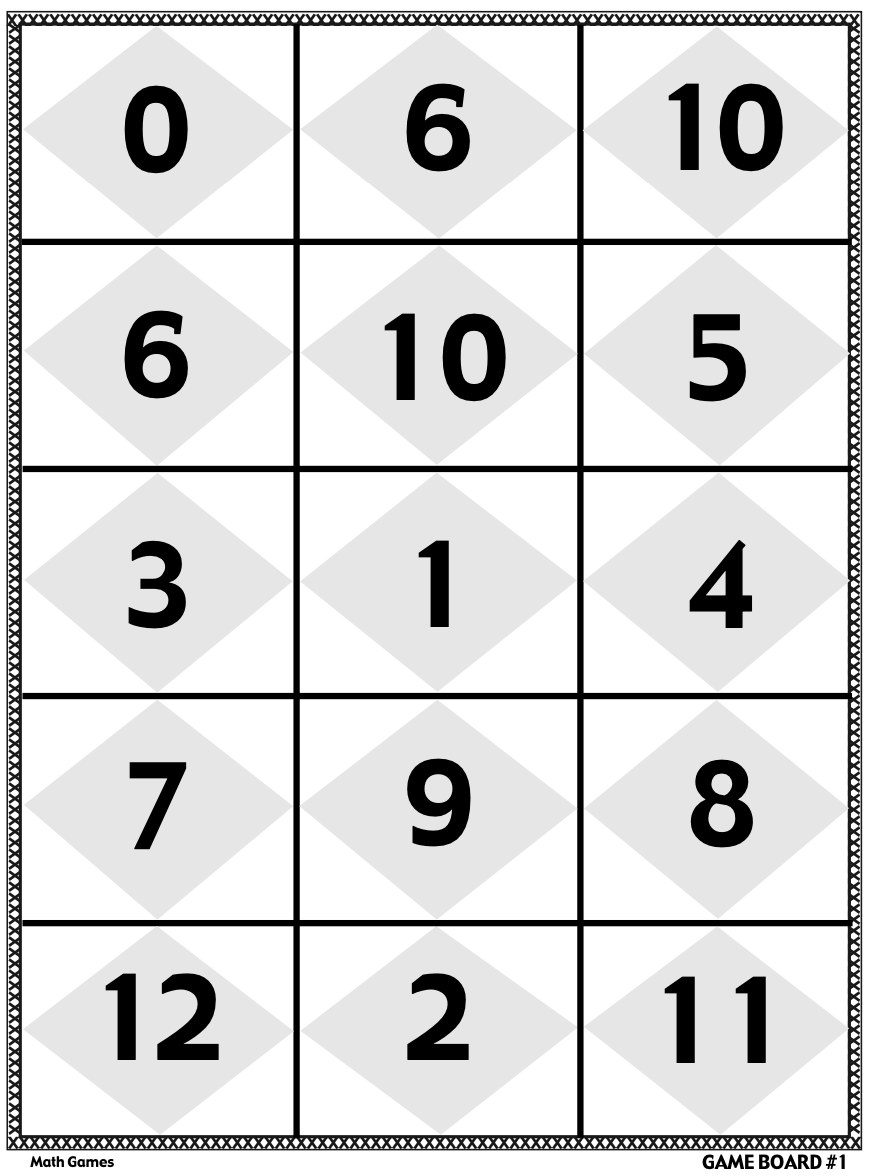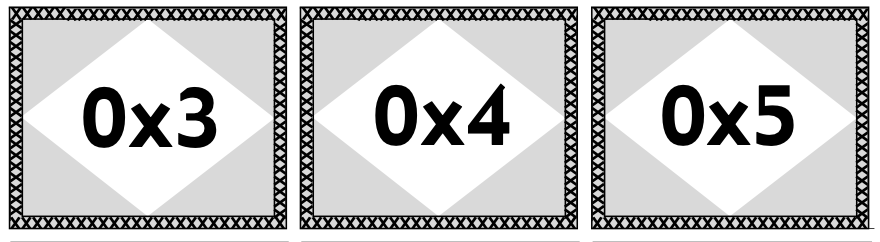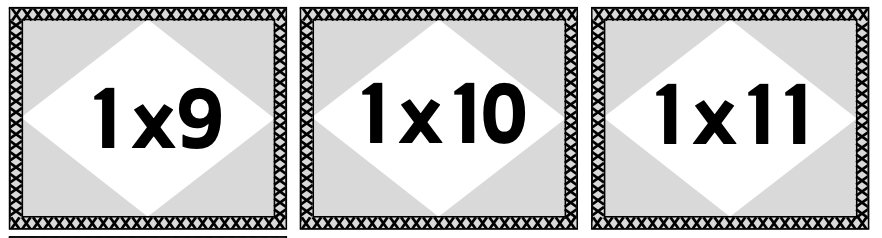# 🌹Make Math Fun with Fact Fluency for ELLs

Hello teachers ~

Today we are going to be diving into all things related to math fact fluency. Fact fluency is a skill that students especially English Language Learners need to develop in order to be successful with math. This post will unpack what fact fluency is, any misconceptions, activities to teach it, and how to make it work for your ELLs. Let’s dive in! 😁

_____________________________________________________

⭐What is math fact fluency?

💧What is 6 x 1?

💧What is 5 x 2?

💧What is 4 x 3?

If you are able to recall the answer to these questions in 2 seconds or less, you may have developed something called math fact fluency

In simple terms, math fact fluency occurs when a student instantly recalls the answer to a basic math problem. As students repeatedly practice simple addition, subtraction, multiplication, and division equations, they commit the answers to their long-term memory. When students are able to recall these answers instantly, they have achieved math fact fluency.

However, math fact fluency is also more than that. In order to possess this skill, students need to feel comfortable with numbers and enjoy working with them. Completing multiplication drills is monotonous and boring for students, but participating in fun activities can help them build their math fact fluency in meaningful ways.

____________________________________________________

Math fact fluency vs. Math fact automaticity

The internet is filled with terms related to math facts. Sometimes it’s difficult to know what the difference between them is! Two common terms that can easily be interchanged are math fact fluency and math fact automaticity. Let’s discuss the difference below.

👆Math Fact Fluency – the ability to solve math problems quickly and fluently. Fluency in math refers to being able to manipulate numbers to find an answer quickly. For example, if you posed the question, “how could I solve the problem 3 x 4?” students with fact fluency may know the answer is 12, but their basis in visualizing and manipulating numbers is what has caused them to possess the ability to solve this problem so quickly.

✌Math Fact Automaticity – being able to automatically provide the answer to a question without thinking.

While automaticity is a part of math fact fluency, we want to instill a desire to love math and number manipulation, not just spewing out an answer like a robot.

_____________________________________________________

Lesson Plan

Looking for a way to teach multiplication fact fluency to your students? Take a look at the lesson plan below!

Math fact fluency can only be built once students have foundational skills. To teach students multiplication fact fluency, you must first teach students how to multiply. Great ways to do this include visual activities like arrays, repeated addition, or creating equal groups with manipulatives. These activities will get students’ minds turning with the understanding of how multiplication works. This is vital for students to understand before they move into fact fluency.

Wondering how to build a background for ELL students? Vocabulary is the cornerstone of learning for language learners. Pull a few keywords from the topic and teach them to students before beginning your math unit. This ensures that students know and understand the language needed to interact with the topic. As students learn, play games with students to reinforce the vocabulary. Need help getting started? My math vocabulary bundle in English and Spanish includes keywords and pictures for several topics.

If your students already have a strong foundation of how multiplying works, you can move them into fact fluency. To practice fact fluency, we must give students repetitive, engaging opportunities to practice solving equations.

_____________________________________________________

There are several activities that I LOVE for teaching multiplication fact fluency. Let’s explore one of them together.

For math games and activities, I especially enjoy using flashcards and game boards. We use both for the multiplying game from my Multiplication Fact Fluency Games Bundle that we are going to explore in this lesson.

First, each student takes a game board, like the one below. (I have several printable game boards in my resource.)Next, students take turns drawing an equation from the pile of facedown equation cards in the center of the table. Example equation cards are below:Once students draw their equation cards, they read the equation aloud and try to determine the answer. As they are solving, their group mates try to solve the equation as well to be sure that the student who is answering the question gives the correct answer. Once the student solves the problem, he or she looks to see if the answer is on their game board. If it is, they cover the answer with the equation card itself. If it isn’t, they put the equation card in the discard pile. Play passes to the next player. The game ends when a player has filled his or her entire board with equation cards.

Students love playing this game because they have the opportunity to compete against their group mates to fill up their board first! What student doesn’t love trying to be the first to win? This game helps build math fact fluency because students are practicing math in a meaningful way. Students are engaged as they repeatedly attempt to solve math equations with their group mates.

Have ESL students in your class? Because fact fluency is primarily number-based, the skill itself requires little language. As long as students know the basic vocabulary surrounding the topic, students at all language levels, newcomers included, can participate in these math games with ease. This is also a great unit to have newcomer students practice number vocabulary in English during small group time.

Get this game and many others in my multiplication bundle. This resource also contains timed tests and other multiplication worksheets and activities. Covering numbers 0-12, this unit is all you need to keep your students engaged during math small group, whole group, or as extra homework practice within your multiplication unit!

Your students are right around the corner from achieving math fact fluency. Let this resource help them practice in a meaningful way!

_____________________________________________________

Click the pictures below to take a peek at my resources. 👀

Happy Teaching! 💜